Next: Choice of the Force Up: Results Previous: Results   Contents

## Slow-response Quenched Velocity Verlet

In practice we find that the value of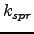affects the convergence properties and the stability of the optimisation process. This result depends on the type of minimiser employed and may also depend on minimiser-specific settings. Here we analyse the convergence properties of NEB minimisations using the QVV minimiser (NEB/QVV) and their dependence on the type of velocity quenching. From previous work it is not clear when is the best time to perform quenching during the MD minimisation of the NEB [29,28,27]. Since the VV algorithm calculates velocities based on the gradients at both current and previous steps quenching could be applied using either of these gradients.

Specifically, it is possible to quench velocities right after advancing the system using Equation 2.14, at the half-step in the velocity evaluation (quenching intermediate velocities at time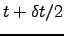) using either the old or new gradient [Equation 2.15], or after completion of the velocity update. In Figure 2.4 we present results for the stability of NEB/QVV as a function of the force constant parameter for three of these quenching approaches. We will refer to an NEB optimisation as stable for a certain combination of parameters (e.g. time integration step, number of images) if the NEB steadily converges to a well-defined path and/or stays in its proximity until the maximal number of iterations is reached or the convergence criterion is satisfied.

We have performed some of the tests on the Müller-Brown (MB) two-dimensional surface . This widely used surface does not present a very challenging or realistic test case, but if an algorithm does not behave well for this system it is unlikely to be useful. MB potential is defined as a sum of four terms, each of which takes the form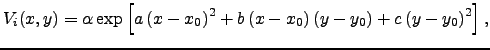(4.35)

where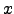and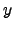are variables and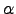,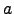,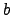,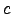,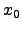and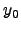are parameters. In the form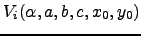these parameters can be written down as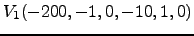,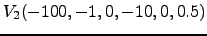,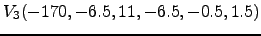and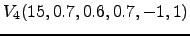. A contour plot of Müller-Brown surface is depicted in Figure 2.3.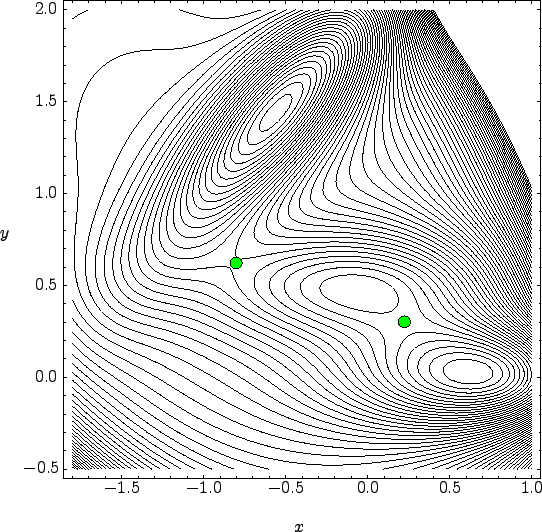Figure 2.4 shows the results of several thousand optimisations for a 17-image band with the MB two-dimensional potential  using QVV minimisation and a time step of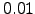(consistent units) for different values of.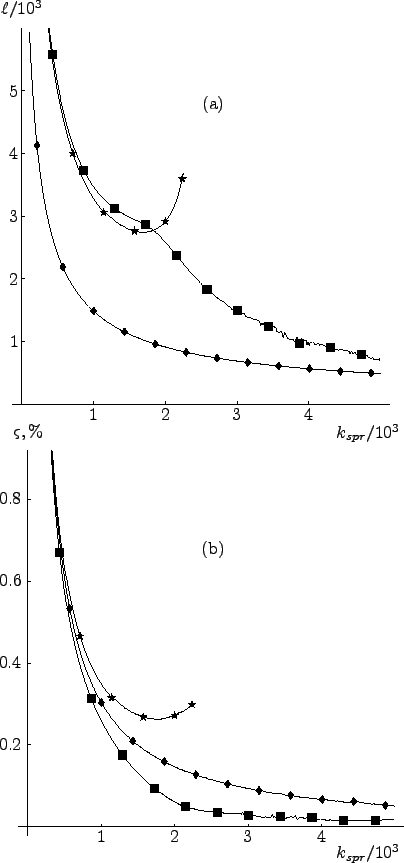Each run was started from the initial guess obtained using linear interpolation and terminated when the root-mean-square (RMS) gradient became less than. We define the RMS gradient for the NEB as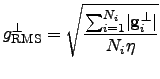(4.36)

where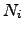is the number of images in the band and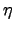is the number of atomic degrees of freedom available to each image.

It seems natural to remove the velocity component perpendicular to the gradient at the current point when the geometry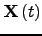, gradient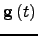and velocity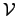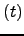are available, i.e.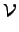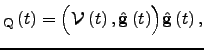(4.37)

where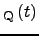is the velocity vector after quenching. However, we found this approach to be the least stable of all -- the optimisation was slow and convergence was very sensitive to the magnitude of the time step. Hence we do not show any results for this type of quenching.

From Figure 2.4 (a) we see that the best approach is to quench the velocity after the coordinate update. The optimisation is then stable for a wide range of force constant values, and the images on the resulting pathway are evenly distributed. In this quenching formulation the velocity response to a new gradient direction is retarded by one step in coordinate space: the step is still taken in the directionbut the corresponding velocity component is removed. To implement this slow-response QVV (SQVV) it is necessary to modify the VV algorithm described in Section 2.5.1 by inserting Equation 2.37 in between the two stages described by Equation 2.14 and Equation 2.15.

The second-best approach after SQVV is to quench the velocity at midstepusing the new gradient. On average, this algorithm takes twice as long to converge the NEB to a given RMS gradient tolerance compared to SQVV. However, the method is stable for the same range of spring force constant values and produces a pathway in which the images are equispaced more accurately than the other formulations [see Figure 2.4 (b)].

The least successful of the three QVV schemes considered involves quenching velocities at mid-step using the gradient from the previous iteration (stars in Figure 2.4). Even though the number of iterations required is roughly comparable to that obtained by quenching using the new gradient, it has the smallest range of values for the force constant where it is stable. Some current implementations of NEB [137,136] (intended for use in combination with electronic structure codes) use this type of quenching in their QVV implementation.

We have also conducted analogous calculations for more complicated systems such as permutational rearrangements of Lennard-Jones clusters. The results are omitted for brevity, but agree with the conclusions drawn from the simpler 2D model described above. The same is true for the choice of force constant investigated in the following section.Next: Choice of the Force Up: Results Previous: Results   Contents
Semen A Trygubenko 2006-04-10